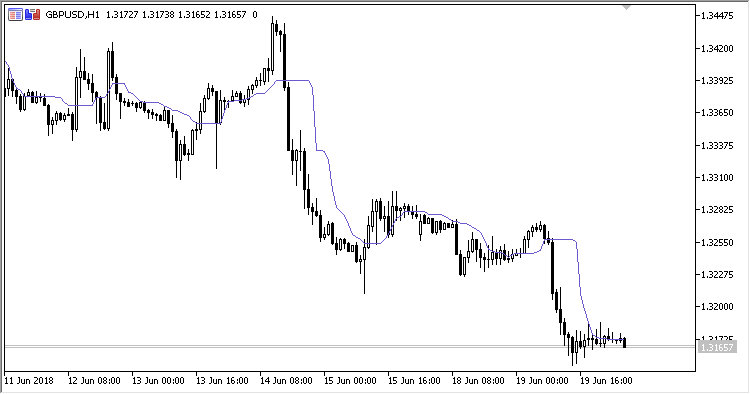Interesting script?
So post a link to it -
let others appraise it

You liked the script? Try it in the MetaTrader 5 terminal# RMI - indicator for MetaTrader 5

Views:
1247
Rating:
Published:
2018.07.13 15:21

Indicator RMI (Running Median Indicator) is a moving average with the "Running Median" filtering algorithm.

It has two configurable parameters:

• Period - calculation period;
• Applied price - price used for calculations.

Calculations:

`RMI = VAR(Index)`

where:

• VAR - the values of Applied price in ascending order within Period.
`Index = floor(Length/2.0+0.5)`

If round(Period/2) * 2 = Period:

`Length = Period + 1`

Otherwise:

`Length = Period`Translated from Russian by MetaQuotes Software Corp.
Original code: https://www.mql5.com/ru/code/21166PVO

Indicator PVO (Percentage Volume Oscillator) represents a momentum for the volume. It calculates the difference in the percents of large MA between two moving averages based on volumes.MPO

Indicator MPO (Midpoint Oscillator) displays price movement without its trend component around its centerpoint. It is displayed as two lines: Price movement line and signal line. Centerline (MidPoint) is zero level of the oscillator.SS

Indicator SS (Stochastic Stack) represents a set of eight indicators Stochastic with different parameters, united in a single calculation process.UDP

Indicator UDP (Up/Down Percentage) displays the direction of candlesticks, i.e., up/down, as a percentage over the selected period.